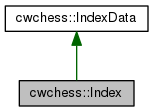cwchess::Index Class Reference

The index of a chess square. More...

`#include <Index.h>`

Inheritance diagram for cwchess::Index:[legend]

## Public Member Functions

Constructors
Index (void)
Construct an uninitialized Index object.

Index (Index const& index)
Copy-constructor.

Index (IndexData index)
Construct an Index object from a constant.

Index (int col, int row)
Construct an Index for column col and row row.

Assignment operators
Index&  operator= (Index const& index)

Index&  operator= (IndexData index)

Accessors
int row (void) const
Returns the row.

int col (void) const
Returns the column.

uint8_t operator() (void) const
Return the unlaying integral value.

Special functions
Advance the index to the next bit that is set in mask. More...

Retreat Index to the previous bit that is set. More...

bool may_call_prev_bit_in (void) const
Return TRUE if index is not index_pre_begin and also not 0.

## Friends

Comparision operators
bool operator== (Index const& i1, Index const& i2)

bool operator== (Index const& i1, IndexData i2)

bool operator== (IndexData i1, Index const& i2)

bool operator!= (Index const& i1, Index const& i2)

bool operator!= (Index const& i1, IndexData i2)

bool operator!= (IndexData i1, Index const& i2)

bool operator< (Index const& index1, Index const& index2)

bool operator< (Index const& index1, IndexData const& index2)

bool operator< (IndexData const& index1, Index const& index2)

bool operator<= (Index const& index1, Index const& index2)

bool operator<= (Index const& index1, IndexData const& index2)

bool operator<= (IndexData const& index1, Index const& index2)

bool operator> (Index const& index1, Index const& index2)

bool operator> (Index const& index1, IndexData const& index2)

bool operator> (IndexData const& index1, Index const& index2)

bool operator>= (Index const& index1, Index const& index2)

bool operator>= (Index const& index1, IndexData const& index2)

bool operator>= (IndexData const& index1, Index const& index2)

## Manipulators

Index const&  operator+= (int offset)

Index const&  operator-= (int offset)

Index&  operator++ (void)

Index operator++ (int)

Index&  operator-- (void)

Index operator-- (int)

Index operator+ (Index const& index, int offset)

Index operator+ (int offset, Index const& index)

Index operator- (Index const& index, int offset)

Index operator- (int offset, Index const& index)Protected Attributes inherited from cwchess::IndexData
uint8_t M_bits
00RRRCCC, where RRR is the row and CCC the column.

## Detailed Description

The index of a chess square.

This class represents the index to a square on the chessboard. It's value runs from 0 till 63, where 0 corresponds to a1 and 63 to h8. In addition there are two out of band values: index_pre_begin (255) and index_end (64).

The three least significant bits represent the column, which runs from 0 to 7. The next three bits represent the row, which also runs from 0 to 7.

Hence column 0 corresponds to file a, column 1 to file b etc, while row 0 corresponds to rank 1, row 1 to rank 2 etc.

IndexData, index_pre_begin, index_begin, index_end,

Definition at line 211 of file Index.h.

## Member Function Documentation

 void cwchess::Index::next_bit_in ( uint64_t mask )
inline

Advance the index to the next bit that is set in mask.

Index may be 0xff, in which case it will be set to the first bit that is set in the mask (0...63) if any, or 64 if no bit is set.

Otherwise Index must be in the range [0, 63], in which case a value is returned larger than the current value. If no more bits could be found, Index is set to 64.

Parameters

Definition at line 360 of file Index.h.

References cwchess::IndexData::M_bits.

 void cwchess::Index::prev_bit_in ( uint64_t mask )
inline

Retreat Index to the previous bit that is set.

Index may be 64, in which case it will be set to the last bit that is set in the BitBoard (0...63) if any, or 0xff if no bit is set.

Otherwise Index must be in the range <0, 63], in which case a value is returned smaller than the current value. If no more bits could be found, Index is set to 0xff.

Normally a value of 0 should always return 0xff, but that is not the case. Don't call this function if the Index is 0.

Parameters